# ELECTRICAL CIRCUIT AND MACHINE ENT 2493 Introduction To

• Slides: 52
Download presentationELECTRICAL CIRCUIT AND MACHINE ENT 249/3 Introduction To Alternating Current and Voltage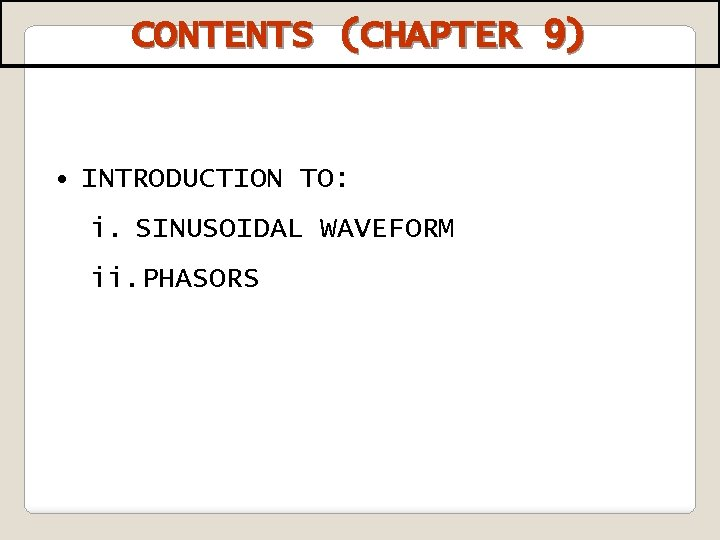CONTENTS (CHAPTER 9) • INTRODUCTION TO: i. SINUSOIDAL WAVEFORM ii. PHASORS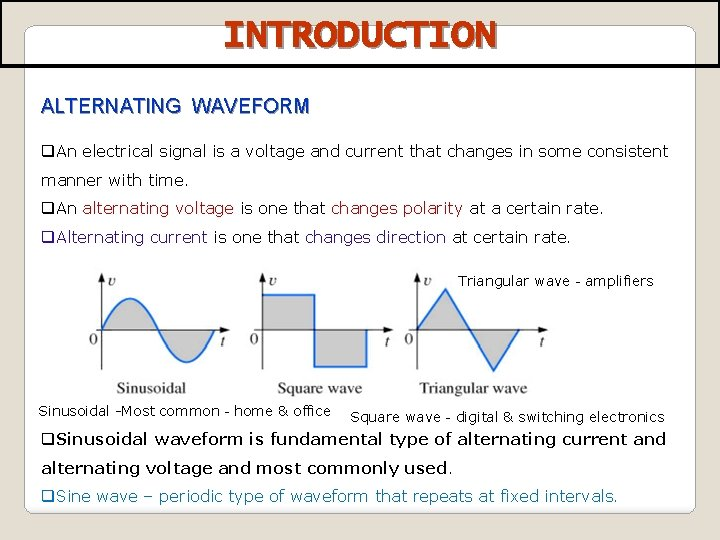INTRODUCTION ALTERNATING WAVEFORM q. An electrical signal is a voltage and current that changes in some consistent manner with time. q. An alternating voltage is one that changes polarity at a certain rate. q. Alternating current is one that changes direction at certain rate. Triangular wave – amplifiers Sinusoidal -Most common – home & office Square wave – digital & switching electronics q. Sinusoidal waveform is fundamental type of alternating current and alternating voltage and most commonly used. q. Sine wave – periodic type of waveform that repeats at fixed intervals.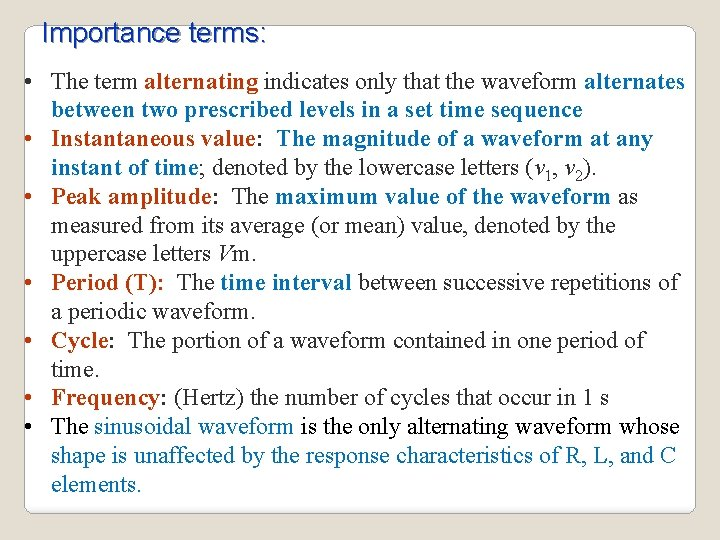Importance terms: • The term alternating indicates only that the waveform alternates between two prescribed levels in a set time sequence • Instantaneous value: The magnitude of a waveform at any instant of time; denoted by the lowercase letters (v 1, v 2). • Peak amplitude: The maximum value of the waveform as measured from its average (or mean) value, denoted by the uppercase letters Vm. • Period (T): The time interval between successive repetitions of a periodic waveform. • Cycle: The portion of a waveform contained in one period of time. • Frequency: (Hertz) the number of cycles that occur in 1 s • The sinusoidal waveform is the only alternating waveform whose shape is unaffected by the response characteristics of R, L, and C elements.SINUSOIDAL WAVEFORM • Produced by two type of source : i. rotating electrical machines (ac generator) ii. Electronic oscillator circuits Symbol of sinusoidal waveform source Graph of one cycle sinusoidal waveform or sine wave Voltage or current varies with time. One cycle of sine wave: i. Starting at zero, voltage or current increase to a positive maximum (peak), return to zero. ii. Then increase to negative maximum (peak) before returning again to zero. ØSine wave changes polarity at its zero value (alternates between positive and negative value. ØCombination of positive and negative alternations make up one cycle of a sine wave.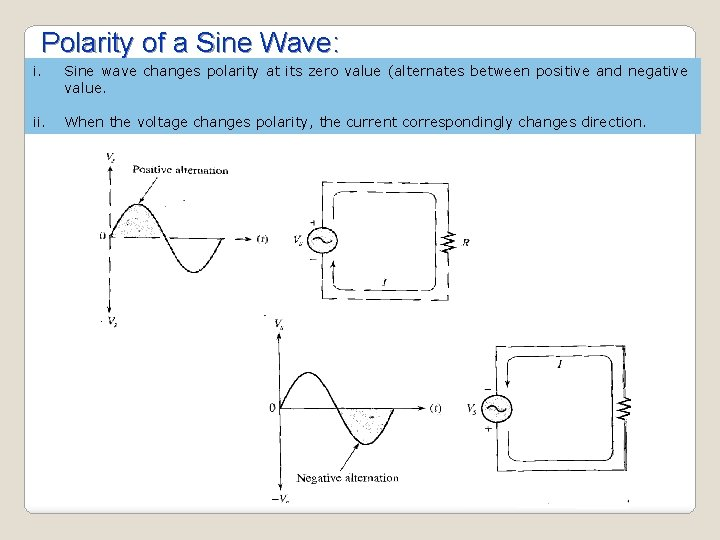Polarity of a Sine Wave: i. Sine wave changes polarity at its zero value (alternates between positive and negative value. ii. When the voltage changes polarity, the current correspondingly changes direction.Period of a Sine Wave: Period (T) - Time required for a sine wave to complete one full cycle Can be measure: i. From a zero crossing to next corresponding zero crossing. ii. From any peak in cycle given to the corresponding peak in the next cycle.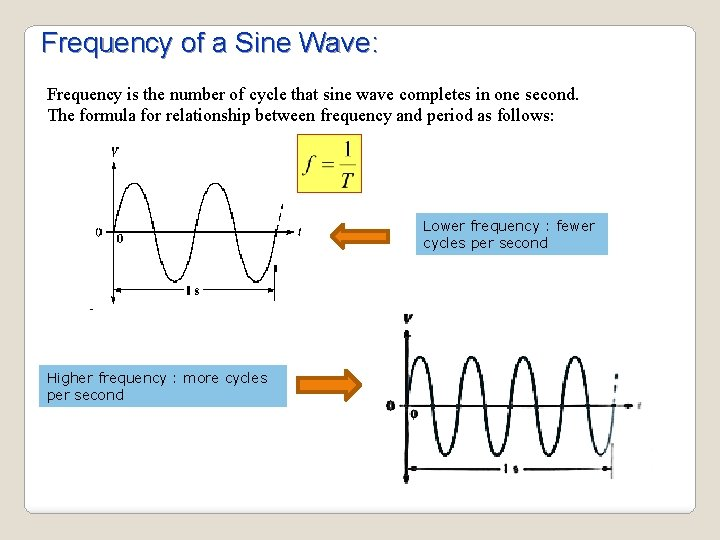Frequency of a Sine Wave: Frequency is the number of cycle that sine wave completes in one second. The formula for relationship between frequency and period as follows: Lower frequency : fewer cycles per second Higher frequency : more cycles per second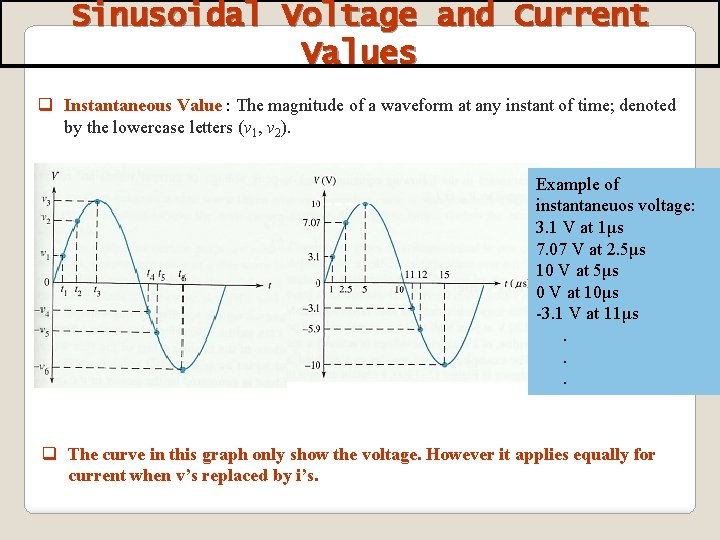Sinusoidal Voltage and Current Values q Instantaneous Value : The magnitude of a waveform at any instant of time; denoted by the lowercase letters (v 1, v 2). Example of instantaneuos voltage: 3. 1 V at 1μs 7. 07 V at 2. 5μs 10 V at 5μs 0 V at 10μs -3. 1 V at 11μs. . . q The curve in this graph only show the voltage. However it applies equally for current when v’s replaced by i’s.q Peak to peak Voltage Vp-p: is the value of twice the peak voltage (amplitude). Peak Value (Vp) = amplitude. Peak Value (Vp) – value of voltage or current at the positive or the negative maximum with respect to zero. q The root-mean-square (RMS) value of a sinusoidal voltage is equal to the dc voltage that produces the same amount of heat in a resistance as does the sinusoidal voltage. q The average value of a sine wave – defined over a half cycle of the wave.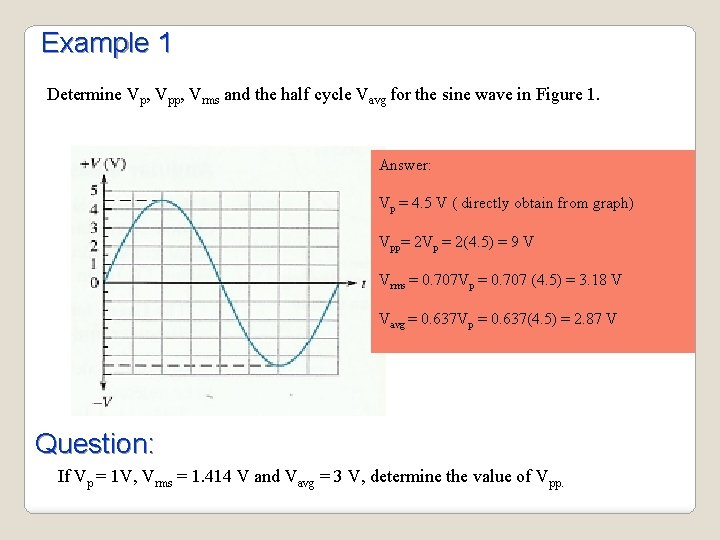Example 1 Determine Vp, Vpp, Vrms and the half cycle Vavg for the sine wave in Figure 1. Answer: Vp = 4. 5 V ( directly obtain from graph) Vpp= 2 Vp = 2(4. 5) = 9 V Vrms = 0. 707 Vp = 0. 707 (4. 5) = 3. 18 V Vavg = 0. 637 Vp = 0. 637(4. 5) = 2. 87 V Question: If Vp = 1 V, Vrms = 1. 414 V and Vavg = 3 V, determine the value of Vpp.Angular Measurement of A Sine Wave ü The sinusoidal voltage can be produced by an alternator, which is an ac generator. ü There is a direct relationship between the rotation of a rotor in an alternator and the sine wave output. ü Thus, the angular measurement of the rotor’s position is directly related to the angle assigned to the sine wave. Ø The sinusoidal wave form can be derived from the length of the vertical projection of a radius vector rotating in a uniform circular motion about a fixed point.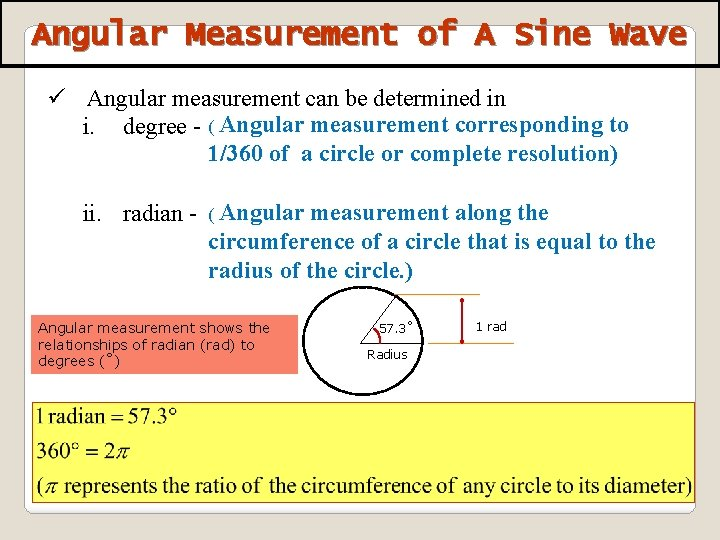Angular Measurement of A Sine Wave ü Angular measurement can be determined in i. degree - ( Angular measurement corresponding to 1/360 of a circle or complete resolution) ii. radian - ( Angular measurement along the circumference of a circle that is equal to the radius of the circle. ) Angular measurement shows the relationships of radian (rad) to degrees (˚) 57. 3˚ Radius 1 radØ The velocity with which the radius vector rotates about the center, called the angular velocity, can be determined from the following equation: The angular velocity ( ) is Ø Since ( ) is typically provided in radians per second, the angle α obtained using α = t is usually in radians.SINUSOIDS Ø The time required to complete one revolution is equal to the period (T) of the sinusoidal waveform. The radians subtended in this time interval are 2π. ØThe basic mathematical format for the sinusoidal waveform is: Vm sin α Ø The equation α = t states that the angle α through which the rotating vector will pass is determined by the angular velocity of the rotating vector and the length of time the vector rotates.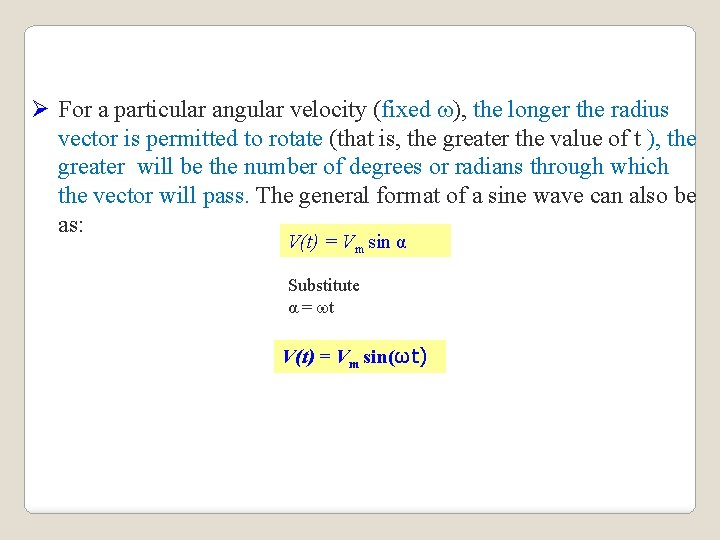Ø For a particular angular velocity (fixed ), the longer the radius vector is permitted to rotate (that is, the greater the value of t ), the greater will be the number of degrees or radians through which the vector will pass. The general format of a sine wave can also be as: V(t) = Vm sin α Substitute α = t V(t) = Vm sin(ωt)SINUSOIDS A sinusoid is a signal that has the form of the sine or cosine function. Ø The sinusoidal current is referred to as alternating current (AC). Circuits driven by AC sources are referred to as AC Circuits. Sketch of Vmsin t. T Period (a) As a function of t. • • (b) As a function of t. Vm is the AMPLITUDE of the sinusoid. is the ANGULAR FREQUENCY in radians/s. f is the FREQUENCY in Hertz. T is the period in seconds.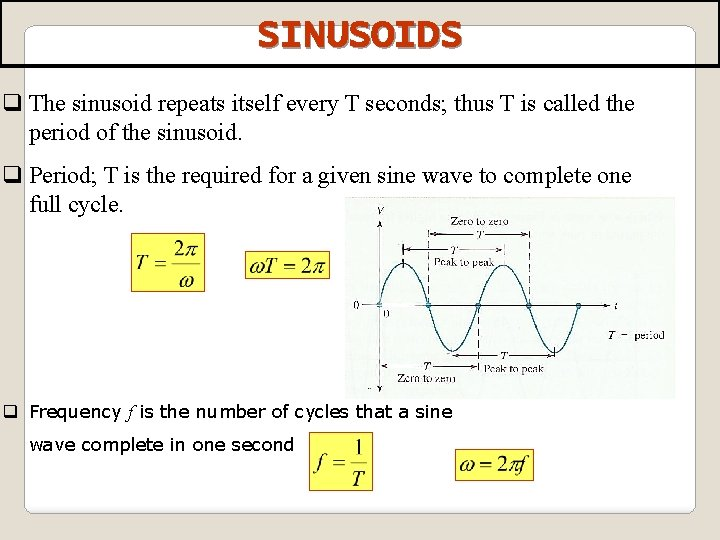SINUSOIDS q The sinusoid repeats itself every T seconds; thus T is called the period of the sinusoid. q Period; T is the required for a given sine wave to complete one full cycle. q Frequency f is the number of cycles that a sine wave complete in one secondPHASE OF SINUSOIDS • The phase of sine wave is an angular measurement that specifies the position of that sine wave relative to a reference. • By referring to this graph, note that the first positive going zero crossing of the horizontal axis (zero crossing) at 0 o, and the positive peak at 90 o. • The negative-going zero crossing at 180 o, and the negative peak at 270 o. • The complete cycle at 360 o. • When the sine wave is shifted to the left or right with respect to this reference, there are phase shift.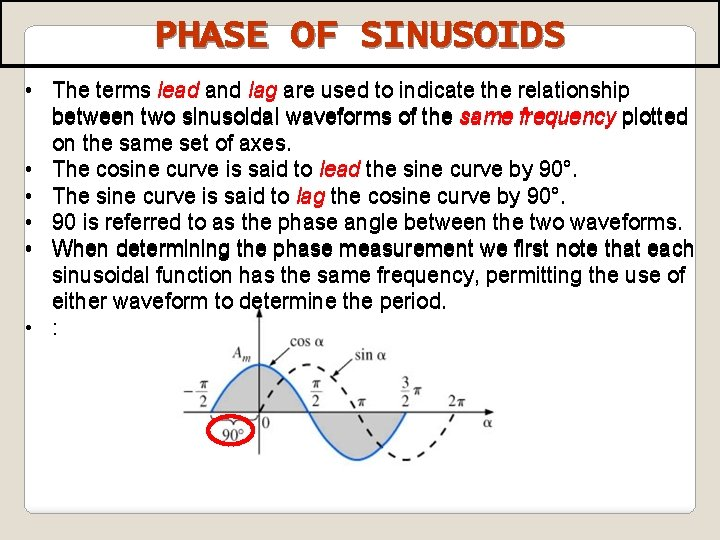PHASE OF SINUSOIDS • The terms lead and lag are used to indicate the relationship between two sinusoidal waveforms of the same frequency plotted on the same set of axes. • The cosine curve is said to lead the sine curve by 90°. • The sine curve is said to lag the cosine curve by 90°. • 90 is referred to as the phase angle between the two waveforms. • When determining the phase measurement we first note that each sinusoidal function has the same frequency, permitting the use of either waveform to determine the period. • :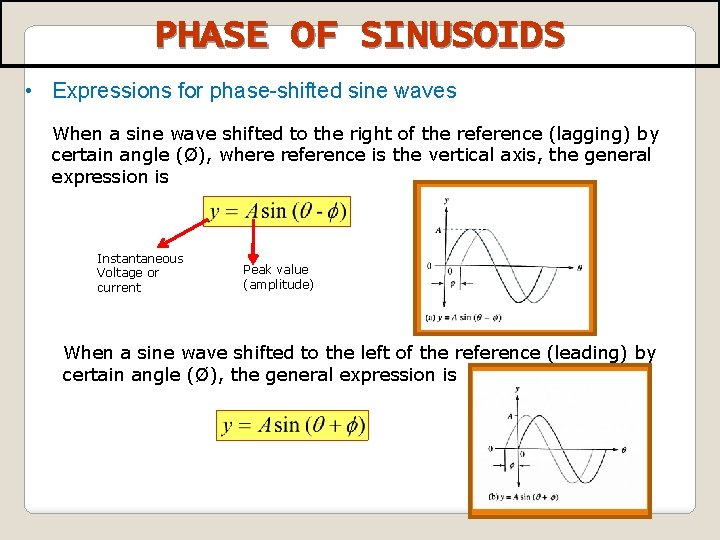PHASE OF SINUSOIDS • Expressions for phase-shifted sine waves When a sine wave shifted to the right of the reference (lagging) by certain angle (Ø), where reference is the vertical axis, the general expression is Instantaneous Voltage or current Peak value (amplitude) When a sine wave shifted to the left of the reference (leading) by certain angle (Ø), the general expression isPHASE OF SINUSOIDS Ø Consider the sinusoidal voltage having phase Ø, V 2 Occur first in time • v 2 LEADS v 1 by phase φ or we can say that v 1 LAGS v 2 by phase φ. • v 1 and v 2 are out of phase by Øo because Ø ≠ 0.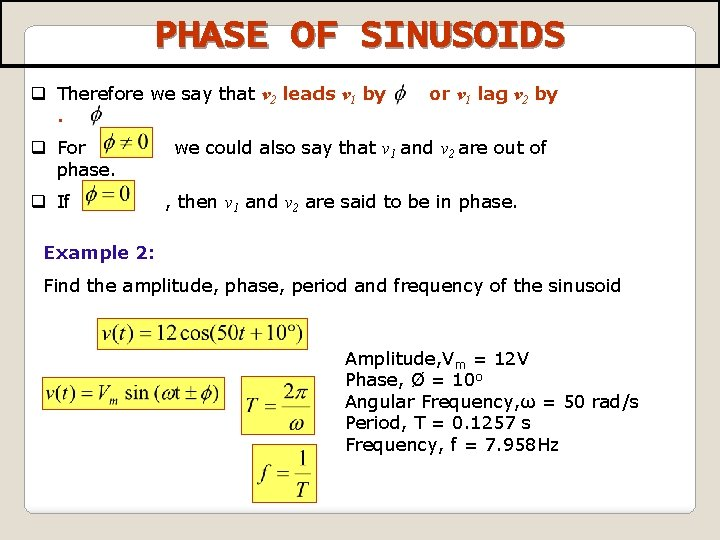PHASE OF SINUSOIDS q Therefore we say that v 2 leads v 1 by. q For phase. q If or v 1 lag v 2 by we could also say that v 1 and v 2 are out of , then v 1 and v 2 are said to be in phase. Example 2: Find the amplitude, phase, period and frequency of the sinusoid Amplitude, Vm = 12 V Phase, Ø = 10 o Angular Frequency, ω = 50 rad/s Period, T = 0. 1257 s Frequency, f = 7. 958 HzExample 2 Given a sinusoid, , calculate its amplitude, phase, angular frequency, period, and frequency. Solution: Amplitude = 5, phase = – 60 o, angular frequency = 4 p rad/s, Period = 0. 5 s, frequency = 2 Hz. 24Question 2: Determine the instantaneous value at the 90 o reference on the horizontal axis for each voltage sine wave in Figure below. Solution : 25Phasor representation of a sine wave Full cycle of a sine wave can be represented by rotation of a phasor through 360˚. The instantaneous value of the sine wave at any poit is equal to the vertical distance from the tip of the phasor to the horizontal axis.PHASE OF SINUSOIDS Question 3: Calculate the phase angle between and. State which sinusoid is leading. Diffrerent form Solution step: i. Since there are 2 different function, make them in the same form either cosine or sine using trigonometric identities. ii. compare the phase angle which one of the voltage leading. Alternative Solution: Compare both using graphical approach or phasor diagram.PHASE OF SINUSOIDS ØSinusoid ca be express in either sine and cosine form. When comparing two sinusoids, it is expedient to express both as either sine or cosine with positive amplitudes. ØUse : Trigonometric Identities: Sine and cosine form conversions : Add two sinusoid of the same frequency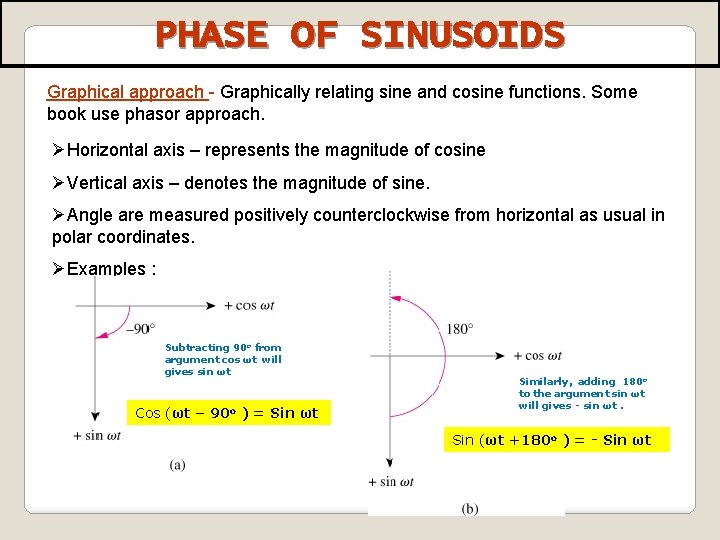PHASE OF SINUSOIDS Graphical approach - Graphically relating sine and cosine functions. Some book use phasor approach. ØHorizontal axis – represents the magnitude of cosine ØVertical axis – denotes the magnitude of sine. ØAngle are measured positively counterclockwise from horizontal as usual in polar coordinates. ØExamples : Subtracting 90 o from argument cos ωt will gives sin ωt Cos (ωt – 90 o ) = Sin ωt Similarly, adding 180 o to the argument sin ωt will gives - sin ωt. Sin (ωt +180 o ) = - Sin ωt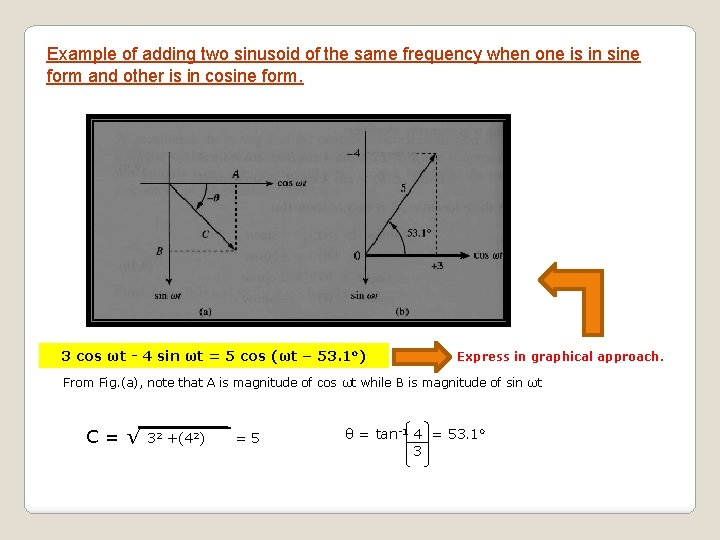Example of adding two sinusoid of the same frequency when one is in sine form and other is in cosine form. 3 cos ωt - 4 sin ωt = 5 cos (ωt – 53. 1 o) Express in graphical approach. From Fig. (a), note that A is magnitude of cos ωt while B is magnitude of sin ωt C=√ 32 +(42) =5 θ = tan-1 4 = 53. 1 o 3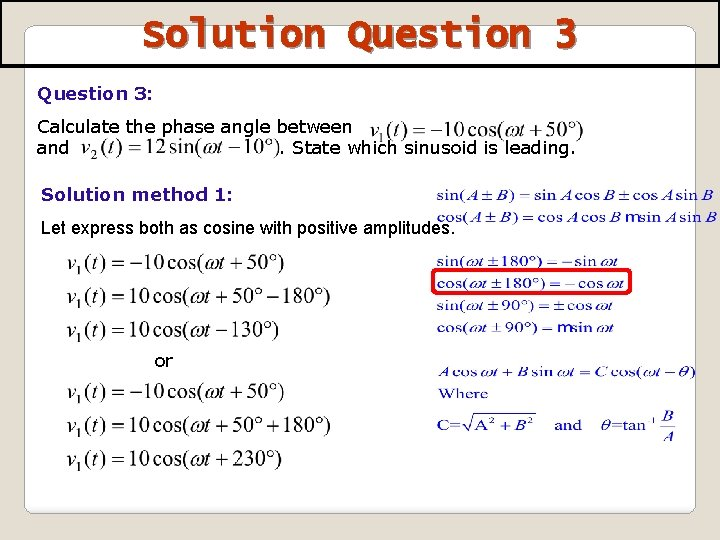Solution Question 3: Calculate the phase angle between and. State which sinusoid is leading. Solution method 1: Let express both as cosine with positive amplitudes. or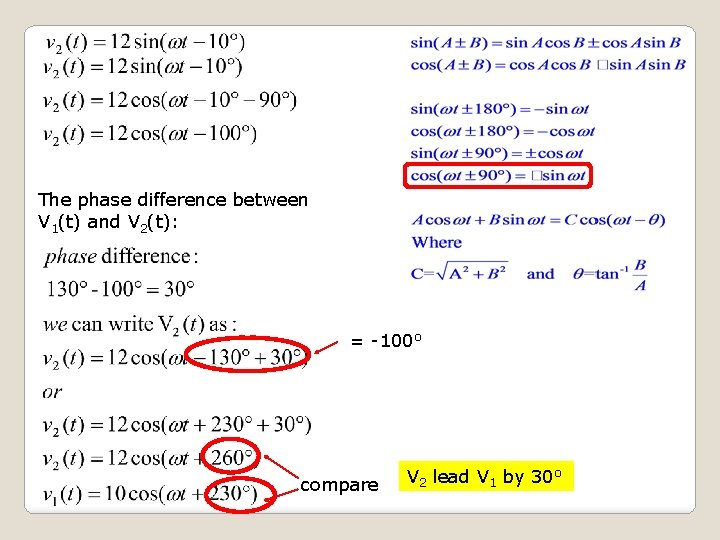The phase difference between V 1(t) and V 2(t): = -100 o compare V 2 lead V 1 by 30 o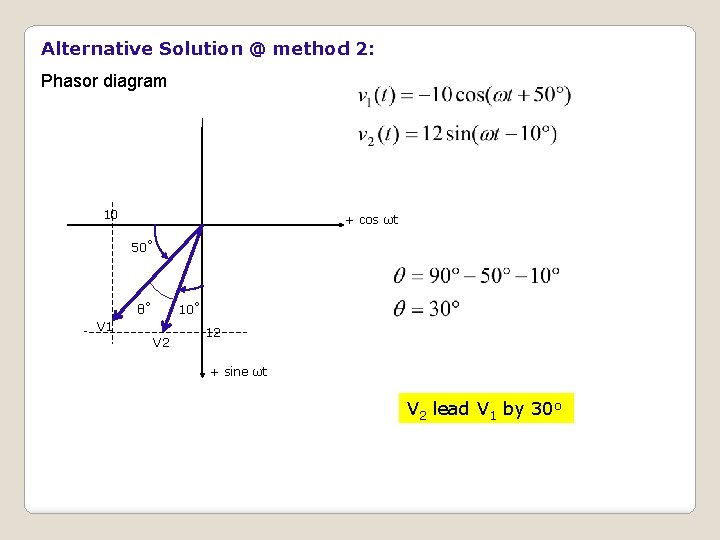Alternative Solution @ method 2: Phasor diagram 10 + cos ωt 50˚ 10˚ θ˚ V 1 V 2 12 + sine ωt V 2 lead V 1 by 30 o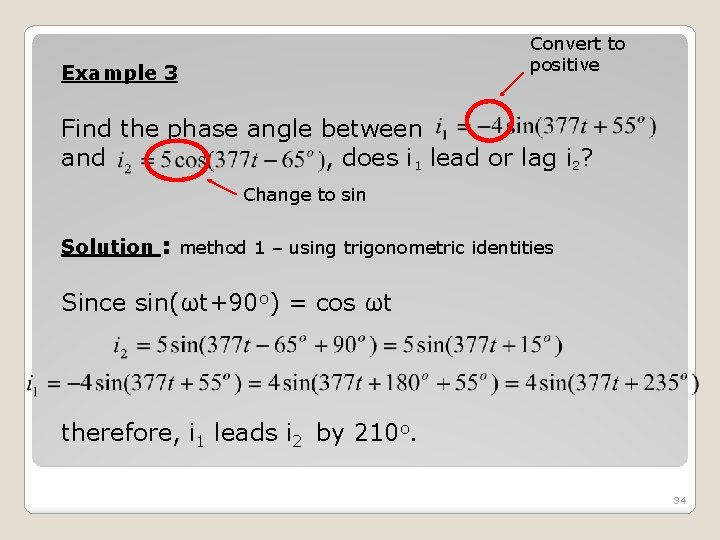Convert to positive Example 3 Find the phase angle between and , does i 1 lead or lag i 2? Change to sin Solution : method 1 – using trigonometric identities Since sin(ωt+90 o) = cos ωt therefore, i 1 leads i 2 by 210 o. 34Alternative Solution : Phasor diagram 4 55˚ i 1 θ˚ + cos ωt 5 65˚ i 2 + sine ωt i 1 lead i 2 by 210 o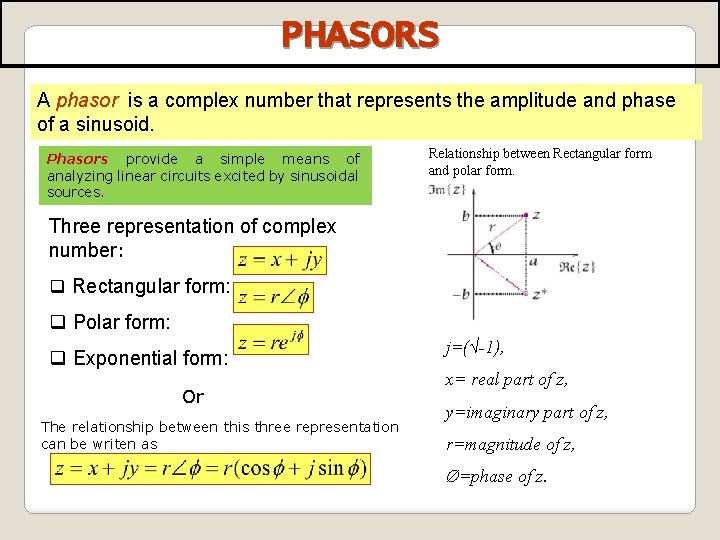PHASORS A phasor is a complex number that represents the amplitude and phase of a sinusoid. Phasors provide a simple means of analyzing linear circuits excited by sinusoidal sources. Relationship between Rectangular form and polar form. Three representation of complex number: q Rectangular form: q Polar form: q Exponential form: Or The relationship between this three representation can be writen as j=(√-1), x= real part of z, y=imaginary part of z, r=magnitude of z, Ø=phase of z.PHASE A complex number may be written in RECTANGULAR FORM asPHASORS We need to convert COMPLEX numbers from one form to the other form.PHASORS Important operations involving complex numbers: q Addition q Subtraction q Multiplication q Division q Reciprocal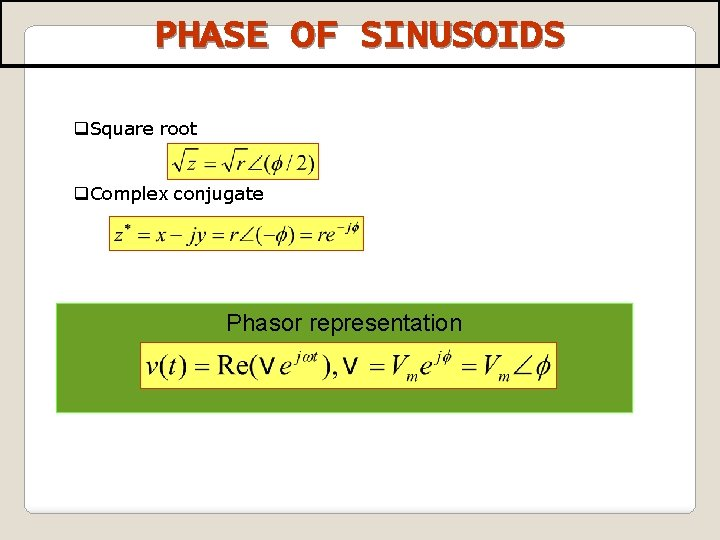PHASE OF SINUSOIDS q. Square root q. Complex conjugate Phasor representationEXAMPLEEXAMPLE 4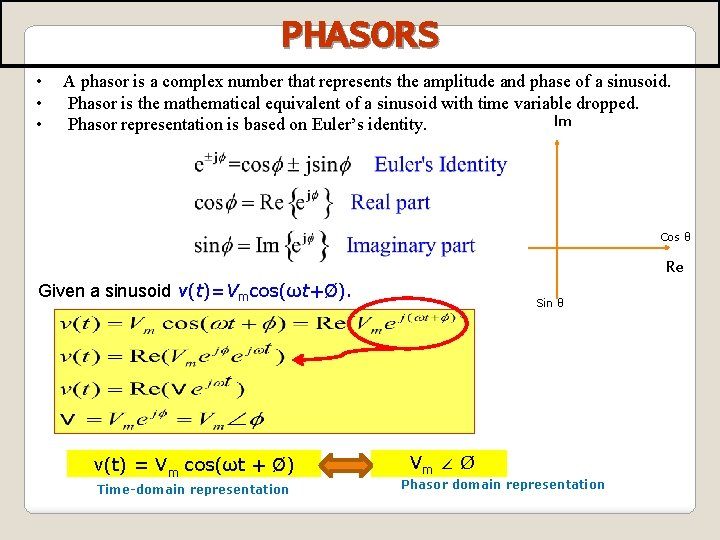PHASORS • • • A phasor is a complex number that represents the amplitude and phase of a sinusoid. Phasor is the mathematical equivalent of a sinusoid with time variable dropped. Im Phasor representation is based on Euler’s identity. Cos θ Re Given a sinusoid v(t)=Vmcos(ωt+Ø). v(t) = Vm cos(ωt + Ø) Time-domain representation Sin θ Vm ∠ Ø Phasor domain representationSinusoid – Phasor Transformation Time domain representation Phasor domain representation Vm cos (ωt + Ø) Vm ∠ Ø Vm sin (ωt + Ø) Vm ∠ Ø – 90 o Im cos (ωt + θ) Im ∠ Ø Im sin (ωt + θ) Im ∠ Ø - 90 o A phasor diagram showing V = Vm ∠Ø and I = Im∠ θ Voltage Current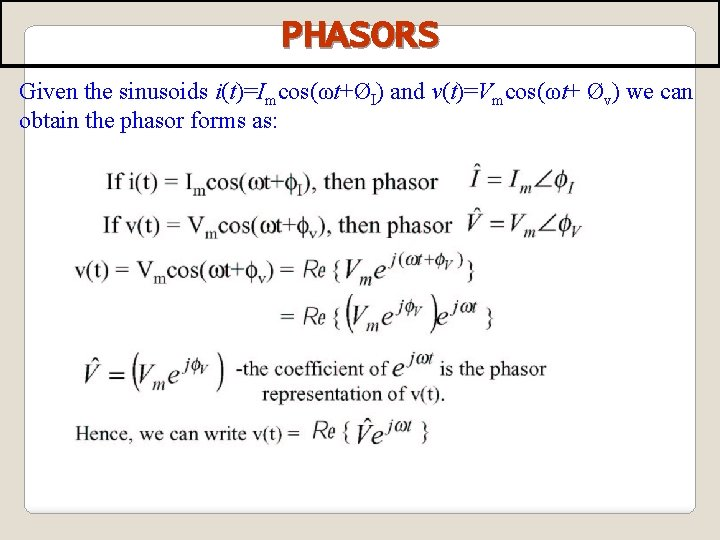PHASORS Given the sinusoids i(t)=Imcos(ωt+ØI) and v(t)=Vmcos(ωt+ Øv) we can obtain the phasor forms as: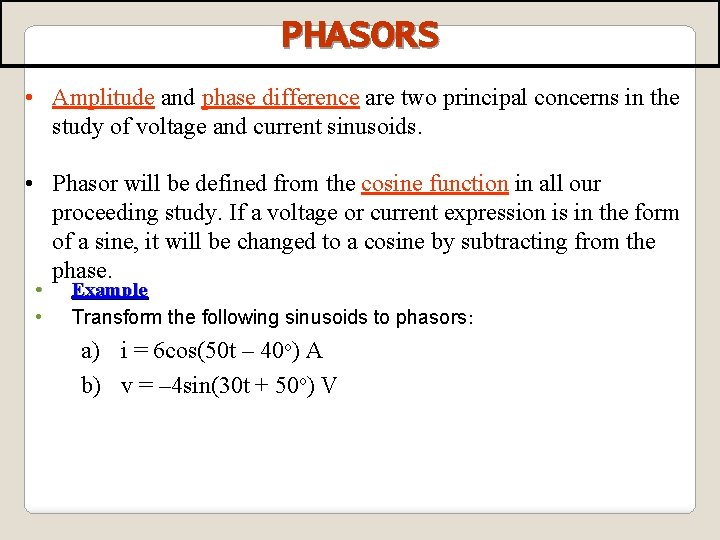PHASORS • Amplitude and phase difference are two principal concerns in the study of voltage and current sinusoids. • Phasor will be defined from the cosine function in all our proceeding study. If a voltage or current expression is in the form of a sine, it will be changed to a cosine by subtracting from the phase. • • Example Transform the following sinusoids to phasors: a) i = 6 cos(50 t – 40 o) A b) v = – 4 sin(30 t + 50 o) VSolution : a. i = 6 cos(50 t – 40 o) A I Im cos (ωt + θ) = Im ∠ Ø A b. v = – 4 sin(30 t + 50 o) V; From the trigonometric identities: –sin(A) = cos(A+90 o); v(t) = 4 cos (30 t+50 o+90 o) = 4 cos(30 t+140 o) V Transform to phasor => V V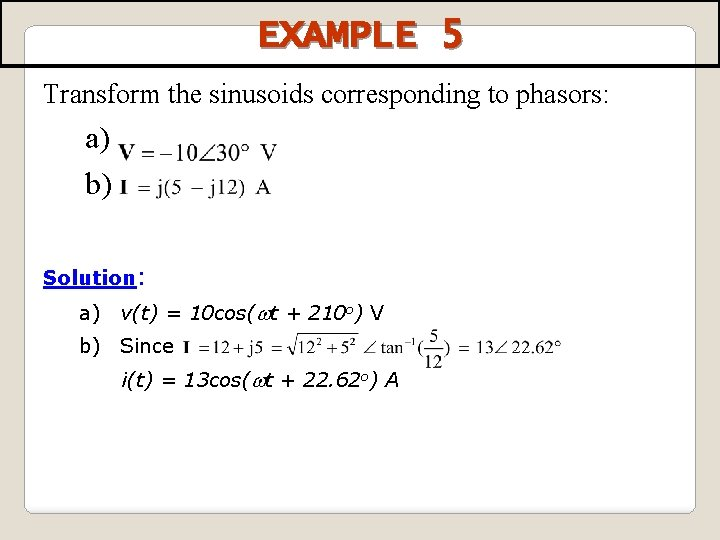EXAMPLE 5 Transform the sinusoids corresponding to phasors: a) b) Solution: a) v(t) = 10 cos(wt + 210 o) V b) Since i(t) = 13 cos(wt + 22. 62 o) A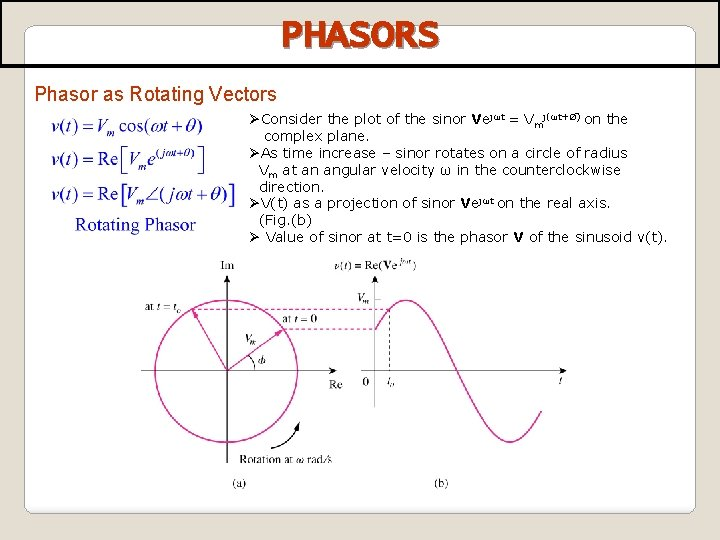PHASORS Phasor as Rotating Vectors ØConsider the plot of the sinor Vejωt = Vmj(ωt+Ø) on the complex plane. ØAs time increase – sinor rotates on a circle of radius Vm at an angular velocity ω in the counterclockwise direction. ØV(t) as a projection of sinor Vejωt on the real axis. (Fig. (b) Ø Value of sinor at t=0 is the phasor V of the sinusoid v(t).PHASORS Phasor diagram: q The phasors are rotating anticlockwise as indicated by the arrowed circle. A is leading B by 90 degrees. q Since the two voltages are 90 degrees apart, then the resultant can be found by using Pythagoras, as shown. .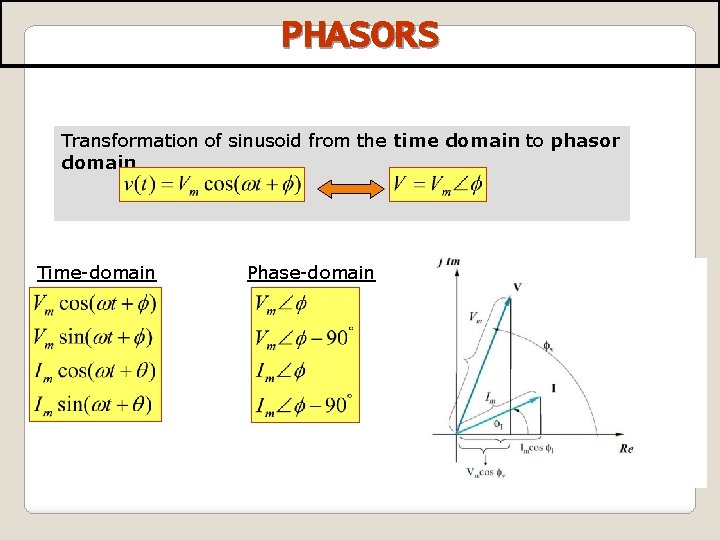PHASORS Transformation of sinusoid from the time domain to phasor domain Time-domain Phase-domain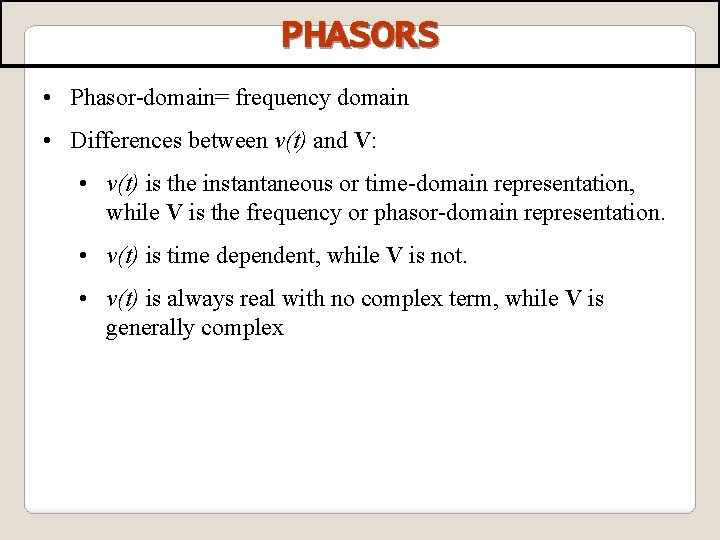PHASORS • Phasor-domain= frequency domain • Differences between v(t) and V: • v(t) is the instantaneous or time-domain representation, while V is the frequency or phasor-domain representation. • v(t) is time dependent, while V is not. • v(t) is always real with no complex term, while V is generally complex